# Kerala Syllabus 9th Standard Maths Solutions Chapter 1 Area

## Kerala State Syllabus 9th Standard Maths Solutions Chapter 1 Area

### Kerala Syllabus 9th Standard Maths Area Text Book Questions and Answers

Textbook Page No. 11

Question 1.
Draw a triangle of sides 3,4 and 6 centimeters. Draw three different right triangles of the same area.
Draw ∆ ABC having sides 3 cm, 4 cm, and 6 cm.Draw a line parallel to AB through C and a line from B perpendicular to the parallel line, both lines intersect at point E. Now ∆ABE is a right angled triangle. Also draw a line perpendicular to the parallel line through A, which makes a point Don parallel line, join BD. ∆ ABD is a right angled triangle.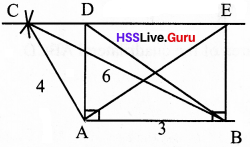Similarly make right-angled triangle by drawing line parallel to AC through B, and a line from A perpendicular to the parallel line, both lines intersect at point F.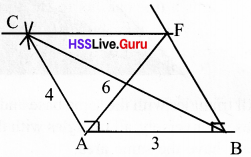The right traingle ∆ DAB, ∆ ABE, ∆ CAF have the same area as that of ∆ ABC.Question 2.
Draw the triangle shown below in your notebook. Draw triangles ABP, BCQ and CAR of the same area with measurements given below.1. ∠BAP = 90°
2. ∠BCQ = 60°
3. ∠ACR = 30°

1.Draw a line parallel to AB through C. Draw a perpendicular from A and the point of intersection of perpen-dicular and parallel line is P. Then draw ∆ABP then ∠BAP = 90°. Areas of ∆ABC and ∆ABP are equal.

2.Draw a line parallel to BC through A from C make angle 60° both lines intersect at point Q. Then draw ∆ BCQ. Areas of ∆ ABC and ∆ BCQ are equal.

3.Draw a line parallel to AC through B from C make angle 30° both lines intersect at point R. Then draw ∆ACR. Areas of ∆ABC and ∆ ACR are equal.

Question 3.
Draw a circle and a triangle with one vertex at the centre of the circle and the other two on the circle. Draw another triangle of the same area with all three vertices on the circle.
Draw a line with centre C and AB is the chord of the circle. Draw ∆ ABC then draw a line parallel to AB through C this line touches the circumference at a point D then complete ∆ ABD. Area of ∆ ABC = Area of ∆ ABDQuestion 4.
How many different (non-congruent) triangles can you draw with two sides 8 and 6 centimetres and area 12 square centimetres? What if the area is to be 24 square centimetres?
For the triangle of area of 12 sq.cm., the height from the 8 cm side should be 3cm.
Then the area = $$\frac{1}{2}$$ x 8 x 3 =12 sq.cm
Draw a line 8 cm long. Draw another line parallel to it at a distance of 3 cm. Draw a circle of radius 6 cm with one end of the first line as the center. The points where this circle cuts the second line are the third vertex of the triangle.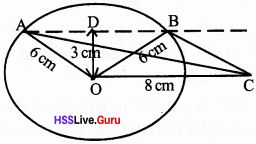We can draw two triangles ∆ OBC and ∆ OCA having area 12 sq.cm.
For the triangle of area 24 sq cm, the height from the 8 cm side should be 6 cm.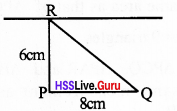We can draw only one triangle of this type.

Question 5.
In the picture below, the lines parallel to each side of the blue triangle through the opposite vertex are drawn to make the big triangle.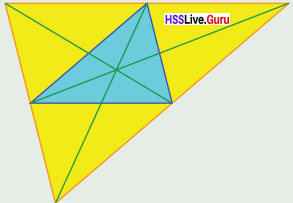How many triangles in the picture have the same area as that of the blue triangle? How many of them have the same measures of the blue triangle?AB is parallel to PQ. So ∆ PAQ and ∆ QPB have the same area as that of ∆ PQR.
BC is parallel to RP, So ∆ CPB and ∆ CRB have the same area as that of ∆ PQR.
AC is parallel to RQ, So A CRA and ∆ CAQ have the same area as that of ∆ PQR.
Also ∆ PCQ, ∆ PAR and ∆ BRQ have the same area as that of ∆ PQR.
Total = 9 triangles
Also ∆ PCQ, ∆ PAR and ∆ BRQ have the same measurement as that of ∆ PQR.
Total = 3 triangles.Question 6.
Prove that the two triangles shown below have the same area:∠ A = 50°
∠B = 130°
∠A + ∠B = 50 + 130 = 180°
These angles are supplementary. So lines AD and BC are parallel. Their lengths are equal.
Since these triangles have the same base and their third vertices on a line parallel to the base, their areas are equal.

Textbook Page No. 15

Question 1.
Draw the two quadrilaterals shown below, in your note book. Draw tri-angles of the same area and calcu-late the areas (The lengths needed may be measured).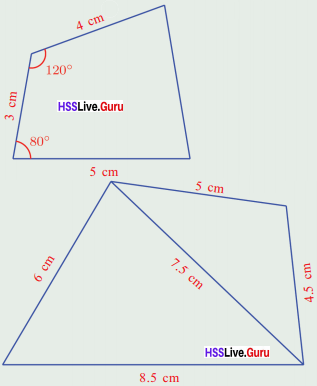1.In the given figure, a line parallel to BD through C and AB produced intersect at E. Now area of ∆AED is equal to the area of quadrilateral. Measure the required lengths and then find the area.
AE = 10.8 cm
Height from D to AE = 2.9 cm
Area of ∆ AED = $$\frac{1}{2}$$ x 10.6 x 2.9
= 15.66 sq.cm

2.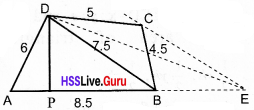In the given figure, a line parallel to BD through C and AB produced intersect at E. Now area of ∆ AED is equal to the area of quadrilateral. Measure the required lengths and then find the area. AE = 12.6 cm DP = 5.2 cm Area of ∆ AED = $$\frac{1}{2}$$ x AE X PD
= $$\frac{1}{2}$$ x 12.6 x 5.2 = 32.76 sq cmQuestion 2.
Draw a rhombus of sides 6 centimetres and one angle 60°; then draw a right triangle of the same area.Draw rhombus ABCD.
∠A = ∠C = 60° and ∠B = ∠D = 120°
Draw diagonal BD. Draw line parallel to BD through C and AB produced intersect at point E. Now area of rhombus is same as the area of ∆ ADE and ∆ ACE. Lines AC bisect ∠A and ∠C.
∴ Angles of ∆ADE is equal to 30°, 60°, 90°
∴ The triangles drawn are right-angled triangles.

Question 3.
Draw a regular pentagon and then a triangle of the same area. Calculate the area.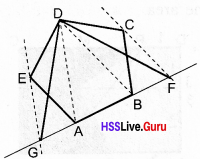Draw a regular pentagon ABCDE. Draw a line parallel to BD through C, line parallel to AD through E, AB produced to both sides intersects points F and G. Now area of AFDG is same as area of pentagon. Measure the required lengths and then find the area.

Question 4.
The picture shows a rectangle divided into two parts. Instead of the broken line separating these parts, draw a straight line to divide the rectangle into two other parts of the same area. Calculate the areas of these parts.Join PQ, draw a line parallel to PQ through O intersecting points are M and N line PN or MQ can make 2 parts with same area.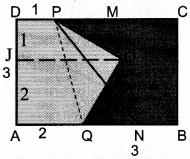Proof:
Area of rectangle ABCD = 5 x 3 = 15 sq.cm
Area of trapezium JOPD = $$\frac{1}{2}$$ x 1 x (1+3) = 2 sq cm
Area of trapezium AQOJ = $$\frac{1}{2}$$ x 2 x (2 + 3) =5 sqcm
Area of part AQOPD = Area of trapezium JOPD + Area of trapezium AQOJ
= 2 + 5 = 7 sqcm
Area of the remaining part = 15 – 7 = 8 sq cm
Now area of trapezium ANPD is equal to the area of pentagon AQOPD.

Textbook Page No. 19

Question 1.
In the picture below, two lines are drawn from the top vertex of a tri-angle to the bottom side:Prove that the ratio in which these lines divide the length of the bot¬tom side is equal to the ratio of the area of the three smaller triangles in the picture.If AB : BC : CD
x : y : z and height = h then area of triangles are $$\frac{1}{2}$$ x h, $$\frac{1}{2}$$ yh, $$\frac{1}{2}$$zh
then ratio of areas are $$\frac{1}{2}$$ xh : $$\frac{1}{2}$$ yh $$\frac{1}{2}$$ zh = x : y : z

Question 2.
In the picture below, the top vertex of a triangle is joined to the mid point of the bottom side of the tri-angle and then the mid point of this line is joined to the other two vertices.Prove that the areas of all four tri-angles obtained thus are equal to a fourth of the area of the whole triangle.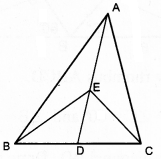Mid point of BC is D.
Line AD divides ∆ ABC into 2 triangles with equal areas.
E is the midpoint of AD.
Line BE divide ∆ ADB into 2 triangles with equal areas. Similarly CE divides ∆ ADC.
4 triangles of equal area.Question 3.
In the picture below, the top vertex of a triangle is joined to the mid point of the opposite side and then the point dividing this line in the ratio 2 :1 is joined to the other two vertices: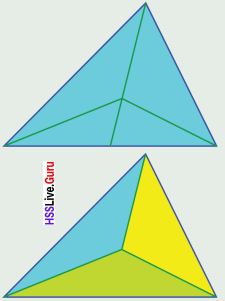Prove that the areas of all three triangles in the picture on the right are equal to a third of the area of the whole triangle.Mid point of AB is Q
∴ ∆ AQC and ∆ BQC have equal area’s P divides AQ in the ratio 2 : 1.
Area of ∆ APC is twice the area of ∆ AQP.
∴ Sum of the areas of ∆ AQP and ∆ BQP is equal to the sum of the area’s of ∆ APC and ∆ BPC.
The area of the APB, PCB and CPA are same. The area of three triangles is equal to the third of area of the whole triangle.

Question 4.
Prove that the lengths of the per-pendiculars from any point on the bisector of an angle to the sides are equal.OC is the bisector of ∠AOB
C ia a point on this bisector.
CA is perpendicular to OA.
CB is perpendicular to OB.
We have to prove that CA = CB Consider the two triangle ∆ OAC and ∆ OBC.
OC = OC (Common side)
∠OAC = ∠OBC = 90°
∠AOC = ∠BOC (OC is the bisector of AOB)
One side and two angles on it of OAC are equal o one side and two angles on it of OBC.
So these triangles are equal.
Then sides opposite to equal angles are equal.
CA = CB
∴ The lengths of the perpendiculars from any point on the bisector of an angle to the sides are equal.Question 5.
In the picture below, the side AC of the triangle ABC is extended to D, by adding the length of the side CB. Then the line through C parallel to DB is drawn to meet AB at E.1. Prove that CE bisects ∠C.
2. Describe how this can be used to divide an 8 centimeters long line in the ratio 4 : 5.
3. Can we use it to divide an 8 centimeters long line in the ratio 3 : 4? How?

1. ∆ BCD is an equilateral triangle If∠CBD = x then ∠CDB = x
∠BCD = 180 – 2x
∠BCE = x
(line BC passing through parallel lines EC and BD makes equal angle)
∴ ∠ACE = x
∴ line CE bisects ∠C.

2. If AB = 8, AC = 4, and CB = 5
then the line CE divides AB in the ratio 4 : 5.

3. No.
We can’t draw a triangle with sides 8cm, 3cm and 4cm. Since 3 : 4 = 6 : 8, take 6cm and 8cm as two sides then find third side.

Question 6.
In the figure, the diagonals of a quadrilateral split it into four tri-angles. The areas of three of them are shown in the picture: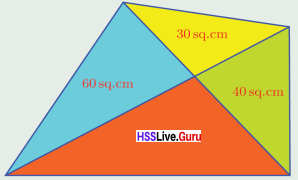Calculate the area of the whole quadrilateral.
A line from the vertex of a triangle divides the length of the opposite side and the area of triangle in the same ratio.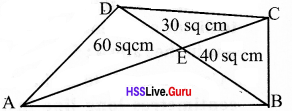In ∆ ACD, a line DE is drawn from D to AC.
∴ AE : EC = Area ∆ AED : Area of ∆ CED
AE : EC = 60 : 30 = 2 : 1
In ∆ ACB, a line BE is drawn from B to AC.
∴ AE : EC = Area of ∆ AEB : Area of ∆ CEB
2 : 1= Area of ∆ AEB : 40 = 2 : 1
∴ Area of ∆ AEB = $$\frac{40×20}{1}$$ =80 sq.cm
∴ Total area of the quadrilateral = 60 + 30 + 80 + 40 = 210 sq.cm

Question 7.
In this picture the hori∠ontal lines at the top and bottom are parallel.Prove that the yellow and red tri-angles are of the same area.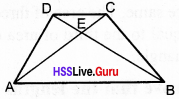AB ∥ DC
∴ Area of ∆ ABC = Area of ∆ ABD (Same base and the third vertex on a line parallel to the base)
Area of ∆ ABC – Area of ∆ ABE = Area of ∆ ABD – Area of ∆ ABE
∴ Area of ABEC = Area of ∆ AED ∆ AED ↔ ∆ BEC

Question 8.
In the figure, the diagonals of a trapezium split it into four triangles.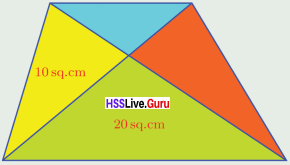The area of the yellow triangle is 10 square centimetres and the area of the green triangle is 20 square centi – metres. What is the area of the whole trapezium?
Since quadrilateral ABCD is a trapezium, lines AB and CD are parallel.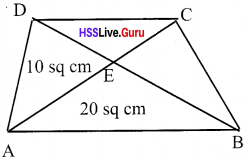So area of ∆ ABD = 20 + 10 = 30 sq.cm
Area of ∆ ABC = 30 sq.cm
(Same base and the third vertex on a line parallel to the base)
Area of ∆ ABC = 30 sq.cm
Area of ∆ ABE = 20 sq.cm
Area of ∆ BEC = 30 – 20 = 10 sq.cm
From ∆ ABC,
AE : EC = area of ∆ ABE : Area of ∆ BEC
AE : EC = 20 : 10 = 2 : 1
From ∆ ACD,
AE : EC = 10 : area of ∆ DEC 2 : 1 = 10: area of ∆ DEC
Area of ∆ DEC = $$\frac{1×10}{2}$$ sq.cm
Total area of the trapezium
= 20 + 10 + 10 + 5
= 45 sq.cmQuestion 9.
The picture below shows a trapezium divided into four parts by the diagonals.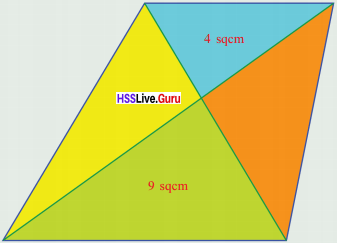The area of the blue triangle is 4 square centimetres and the area of the green triangle is 9 square centimetres. What is the total area of the trapezium?Area of ∆ AMD and ABMC are equal.
If area = A sq. cm then
9 : A = BM : MD
A : 4 = BM : MD;
9 : A = A : 4
A2 = 36
A = 6 sq. cm
∴ Area of trapezium = 6 + 4 + 6 + 9 = 25 sq. cm

### Kerala Syllabus 9th Standard Maths Area Exam Oriented Text Book Questions and Answers

Question 1.
a. Where should be the position P in CD to set the isosceles triangle with half the area of the rectangle.b. Where should be P in CD to get ∠ABP a right angle.
c. If ∠APB is a right angle how can you find the position P?
d. Can such right angled triangle be drawn in any of the rectangle?
a. P is the centre of CD
b. It should be in C.
c. The distance from centre of AB to P should be half the length of AB.
d. No.

Question 2.
The lines in the diagram are parallel. Construct ∆ ABC with ∠C right angled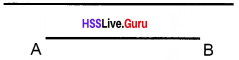AB is the diameter.

Question 3.
Construct ∆ ABC with AB = 8cm, BC = 6cm, AC = 5cm. Draw an isosceles triangle with same area and one side as AB itself.In figure AB ∥ PQ. D is the center point of AB.
Hence ∆ ABD is an isosceles triangle.Question 4.
Find the area of the triangles with the given sides.
(a) 21 cm, 17 cm, 10 cm
(b) 12 cm, 16 cm, 20 cm
(c) 34 cm, 30 cm, 16 cm
(d) 20 cm, 21 cm, 29 cmQuestion 5.
ABCD is a rectangle in the diagram. P is a point on the side CD. Show that the area of ∆ AQB is equal to the sum of the areas of ∆ PQD and ∆ PCB⇒ area of ∆ AQB = area of ∆ APB – area of ∆ BQP
⇒ area of ∆ ADB = area of ∆ DBC area of (∆ ADQ + ∆ AQB) = area of (∆ DQP + ∆ QBP + ∆ BPC ) …….(1)
(∆ DAP = ∆ DBP) ⇒ (∆ ADQ = ∆ QBP) ⇒ area of (∆ ADQ + ∆ AQB)
= area of (∆ DQP + ∆ ADQ + ∆ BPC)
⇒ area of ∆ AQB = area of ∆ DQP + area of ∆ BPC.

Question 6.
In the figure XY, RS are parallel. The area of ∆ ABD is 20 cm2
a) Find the area of the parallelogram ABCD.
b) Find two triangles in the diagram with same area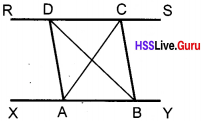a.
20 + 20 = 40 sq. cm
b.
i. ABD, ABC
ii. DCB, DCA

Question 7.
Find the area of triangle in the dia-gram.$$\frac{1}{2}$$ x 6 x 4 = 12 cm

Question 8.
In the figure BP : PQ : QC = 1 : 2 : 1. Area of triangle APQ is 8 sq.cm.
a. Find the area of triangle ABP.
b. Find the area of triangle ABC.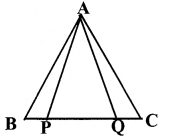a. BP : PQ = 1:2
Area of ∆ ABP is half the area of ∆ APQ.
Area of ∆ ABP = $$\frac{1}{2}$$ x 8 = 4 sq.cm

b. Area of ∆ ABC = 4 + 8 + 4 = 16 sq.cm

Question 9.
In the figure line AB is parallel to CD. If AB = 5cm, AC = 4cm and ∠CAB = 90°.
a. Calculate the area of triangle ABC.
b. How much is the area of triangle ∆ ABD? Write reasona. Area of ∆ ABC = $$\frac{1}{2}$$ x 5 x 4 = 10 sq.cm
b. Area of ∆ ABD = Area of ∆ ABC
Triangles with the same base and the third vertex on a line parallel to the base, have the same area.
Area of ∆ ABD = 10 sq.cmQuestion 10.
Draw triangle ABC with AB = 8cm, BC = 6cm and B = 30°. Draw a right angled triangle of the same area. Measure its perpendicular sides. Calculate the area of the triangle.
Draw ∆ ABC with the given measures.Draw a line through C, parallel to AB.
Mark D on this parallel line such that ∠ BAD = 90°.
∆ ADB is the required triangle.
Measure the required lengths and then find the area.
AB = 8 cm
Area of ∆ ADB = $$\frac{1}{2}$$ x 8 x 2.9 = 11.6 sq.cm

Question 11.
Draw a quadrilateral with given measures. Draw a triangle of the same area as that of the quadrilateral.‘Draw a line through C parallel to DB. Which cuts AB at E.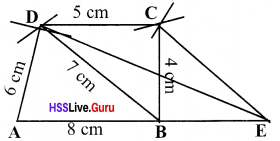∆ AED has the same area as that of quadrilateral ABCD.

Question 12.
If AB = 8cm, AC = 6cm, BC = 9cm,
AD is the bisector of A , then
a. BD : DC = ………….
b. What is the ratio of areas of triangle ABD and triangle ACD?
c. Draw a line of length 9 cm and divide it in the ratio 3 : 4.